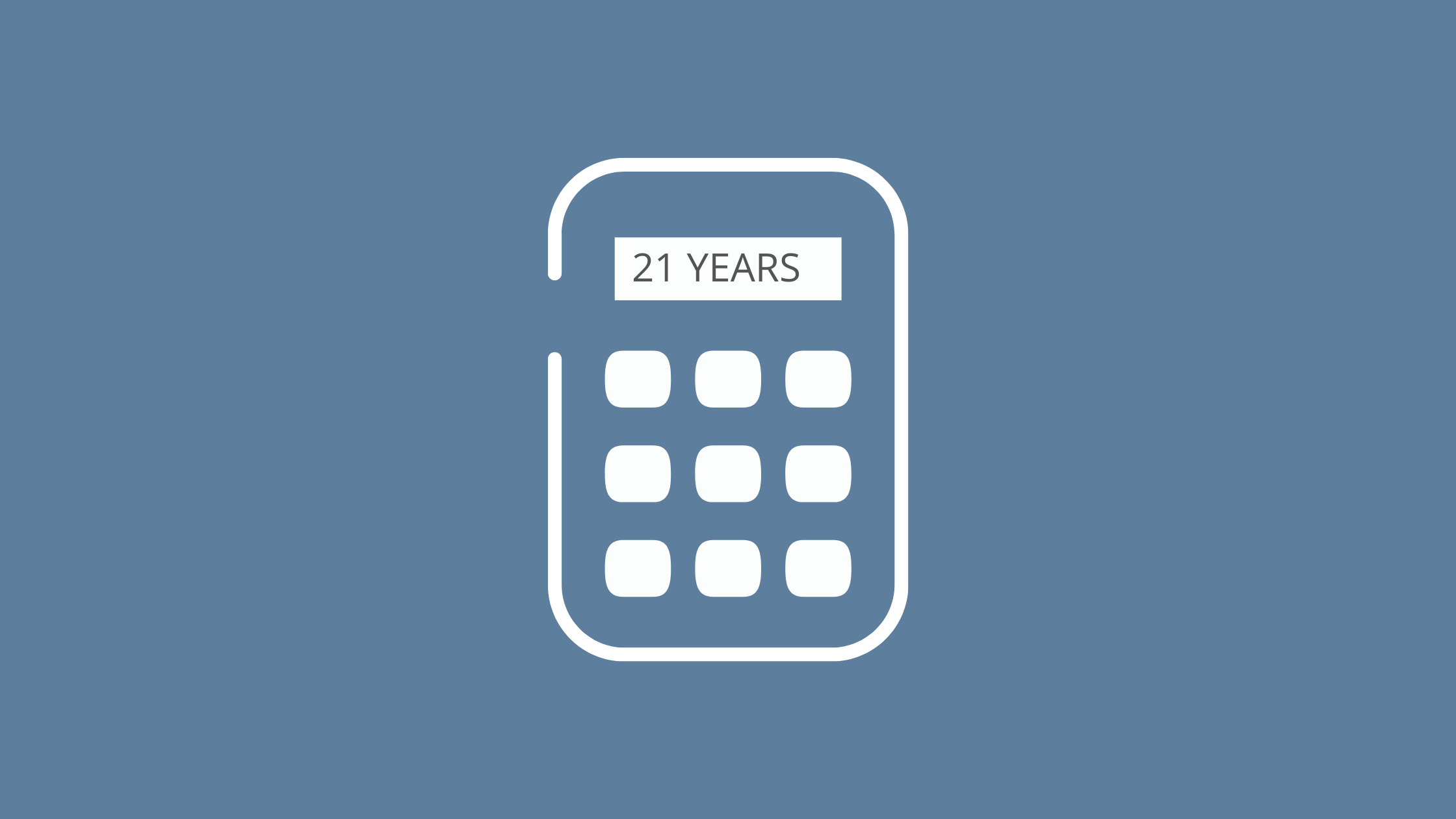Are you looking for “Age Calculator Script In PHP & Javascript Code Free Download” or php calculate age between two dates, age calculator html code w3schools, calculate current age php, php age calculator source code, php calculate age year, month, day, age Looking for calculator source code, age calculator wordpress, simple age calculator in javascript, & javascript age checker, so keep reading this post today.

Age Calculator is an online tool. Which you can use in website and application. This tool calculates the time between any date with its mathematical calculation.

Friends this is a free “Age Calculator Script In PHP & Javascript Code Free Download” or php calculate age between two dates, age calculator html code w3schools, calculate current age php, php age calculator source code, php calculate age year, month, day, Age looking for calculator source code, age calculator wordpress, simple age calculator in javascript, & javascript age checker. And we have made this ourselves. In which we have used HTML, CSS, Jquery, Javascript & PHP.

But we have not designed its UI. Because it is designed for multipurpose. You can use it on WordPress, Wix, and Blogger etc.

Or if you want, you can create your own Ager Calculator website. That too using our Free Age Calculator Script. The demo of which is given below.

# Age Calculator

Advance Age Calculator - Calculate your age in years, months, days, hours, minutes, seconds

Select Date of Birth:

Date :  Month :  Year :

You have been living for:

 In months In days In hours In minutes In seconds Your next birthday will be in: